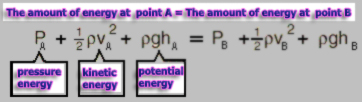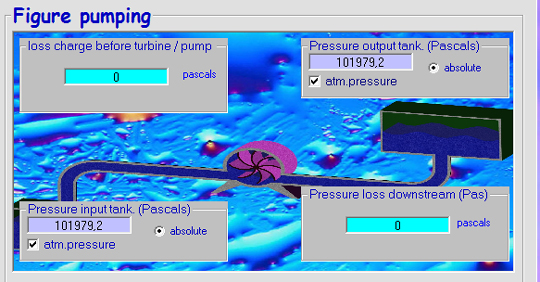The unit of pressure is N/m2 or Pascal (Pa). 1 Pascal represents a force of 1 Newton applied on an area of ​​1 m²: Pressure (pascal) = Force (newton) / Surface(m²)

The pressure generated by a force of 10 N applied to 0.1 m² is equal to the pressure generated by a force of 100 N on 1 m²::

10/0.1=100/1=100 pascals.

This allows to increase the forces depending on pressure surfaces of. (hydraulic cylinders, pressure multipliers ...)

• the absolute pressure (P abs) pressure is measured with reference to a zero pressure (full vacuum).
• Pressure Gauge indicates the difference between the fluid pressure and the atmospheric pressure (P atm).

to convert a value from gauge pressure (PG) to absolute pressure (Pas), simply add the atmospheric pressure:

Pabs = PG + Patm

To convert from absolute pressure to pressure gauge, subtract the atmospheric pressure:

Meteorologists use the pressure in millibars.

1 mb = 0,1 kPa

1 Pa = 1 N/m2, 1 kPa = 1 x 103 Pa

Within a volume of fluid, pressures types are distinguished depending on the physical phenomena that generate them:

• The hydrostatic pressure, or hydrostatic head: This is the pressure generated by the height difference between two points. It represents the force applied by the weight of a fluid column to its base.
• Fluid height (in meters) x density of the fluid (kg/m3) x gravity (9.81) = hydrostatic pressure (in Pascals)

• The dynamic pressure represents the inertia of the fluid, the force produced to generate the acceleration of the fluid mass.

Dynamic pressure (Pascals) = 0.5 x density (kg/m3) x speed² (m / sec)

The sum of the energies of pressure in a same volume of fluid is constant: See bernoulli:The relationship between pressure and flow capacity is very useful to estimate the output power of a fluid system:

• output power Watt = flow (m3/sec) x Pressure (pascals)
• Exemple: a non reversible pressure drop (head loss) of 10 pascals in a 1 m3/sec fluid flow , is a power loss of 10 Watts

The pressure formula : Pressure = Force / Surface is associated in mecaflux to a unit converter.

inMecaflux Standard , the pressures in the ducts are parameterized using the pressure tank (absolute). .To enter a tank pressure other than ambient atmospheric pressure, uncheck the box atm. and enter the absolute pressure.

Attention: reservoir pressures are absolute pressures so if you want to set a gauge pressure (PG), you will have to add the atmospheric pressure (P atm) : Pabs = Patm + PG.

data powers will be updated accordingly ...